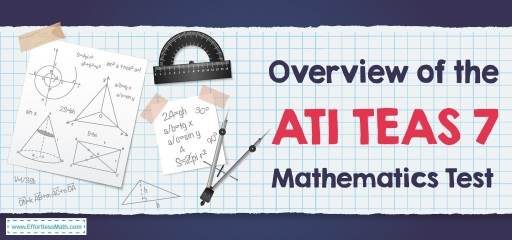# Overview of ATI TEAS 7 Mathematics TestThe Test of Essential Academic Skills or ATI TEAS 7 is a test published in 2016 by the Assessment Technologies Institute (ATI) to assess students’ ability to enter health sciences. The TEAS 7 covers four subjects:

• Reading: 53 Questions – 64 Minutes
• English and Language Usage: 28 Questions – 28 Minutes
• Science: 53 Questions – 63 Minutes
• Math: 36 Questions – 54 Minutes

The ATI TEAS 7 test has 170 questions, all of which are multiple-choice, and you should answer the total questions in 209 minutes.

## What is the structure of the ATI TEAS 7 Mathematics test?

The new math subtest is slightly longer than the TEAS 5 visualization and jumps from 34 to 36 questions. Four questions are not counted in your score and are for test purposes only for future editions. To make up for the increase in questions, you have a little more time to finish – about 54 minutes, instead of the old 51 standards.

The questions on the exam will fall under either Numbers and Algebra or Measurement and Data, as follow:

### 1- Measurement and Data:

The Measurement and Data category has only 9 questions under it. However, it is relatively complex because it deals with quantitative data in various forms.

• Interpret relevant information from charts, tables, and graphs
• Evaluate the information in charts, tables, and graphs using statistics
• Explain the relationship between two variables
• Calculate geometric quantities
• Convert within and between standard and metric systems

Be sure to review both metric and standard units of measurement, as well as arithmetic and other steps related to switching from one system to another. You also need to know the ability of geometric properties and which formulas best apply to which questions, as well as how to properly evaluate the information given to you through graphs, tables, and other similar forms of graphic information.

### 2- Numbers and Algebra:

The average number of questions in the group Numbers and Algebra is 23 questions.

• Convert among non-negative fractions, decimals, and percentage
• Compare and order rational numbers
• Perform arithmetic operations with rational numbers
• Solve equations in one variable
• Solve real-world problems including the percentage
• Solve real-word one-or multi-step problems with rational numbers
• Apply estimation strategies and rounding rules to real-world problems
• Solve real-world problems including proportions
• Translate phrases and sentences into equations, expressions, and inequalities
• Solve real-world problems including ratios and rates of change

To deal with this group of questions as much as you can, you must first strengthen your algebra skills and knowledge. You may want to use rational numbers and their properties, as well as different types of ratios and how to convert them from one to another. Close reading will be especially important because of the large number of word problems in this group. It is you who must pay close attention to the wording of each problem to know what is required of you and how to begin the process of solving it.

## Is the ATI TEAS 7 Mathematics hard?

This test can be as difficult as any other test. But with enough study and practice, you can succeed in this test.

## Do you get a formula sheet on the ATI TEAS 7 Mathematics?

You should know that the math section of ATI TEAS does not provide a list of basic formulas needed to know the test.

## Can you use a calculator on the ATI TEAS 7 Mathematics?

A four-function calculator is provided by the test center. You are not allowed to use the calculators you brought from home.

If you are using the online version of the ATI TEAS test, a drop-down calculator is embedded in the test.

## What score do you need to pass the ATI TEAS 7?

The composite score for the ATI TEAS 7 test is your main score. This score is a combination of your skills in all four sections of the ATI TEAS 7 test. Each school has different admission criteria for your grade, but most schools require at least 60% to 70% of your composite score.

## The Best Books to Ace the ATI TEAS 7 Math Test

### What people say about "Overview of ATI TEAS 7 Mathematics Test - Effortless Math: We Help Students Learn to LOVE Mathematics"?

No one replied yet.

X
52% OFF

Limited time only!

Save Over 52%

SAVE \$40

It was \$76.99 now it is \$36.99